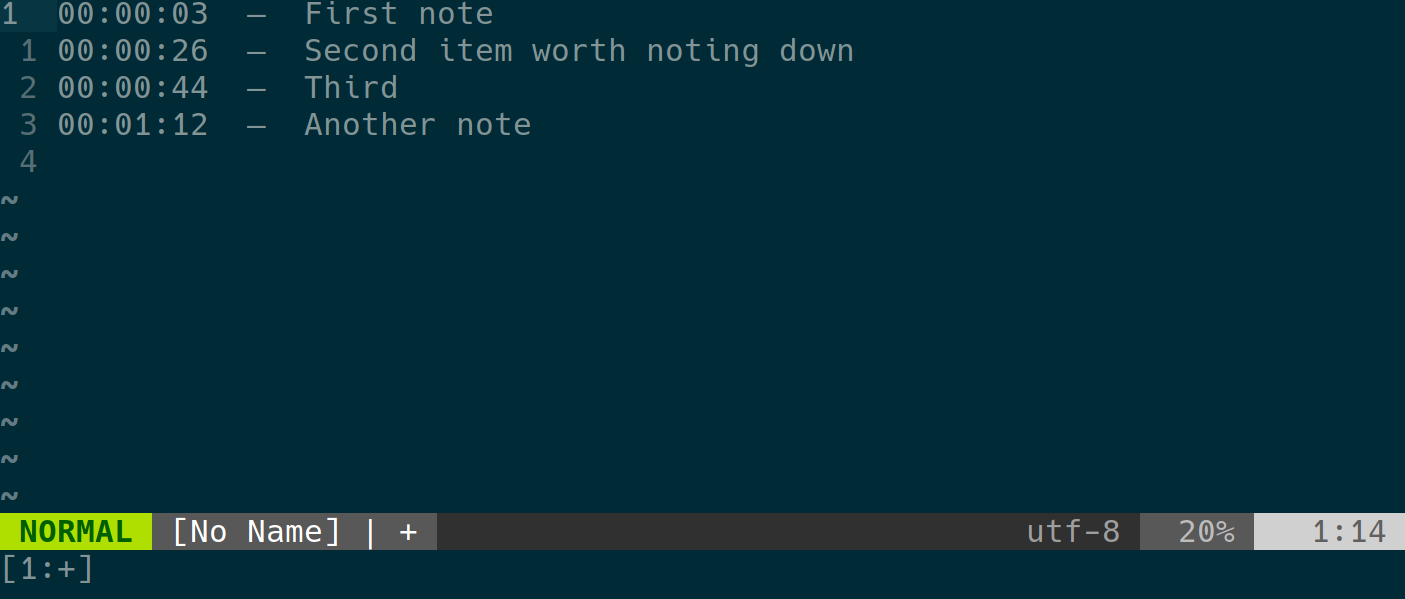## Using Vim to take time-stamped notes

I frequently find myself needing to take time-stamped notes. Specifically, I'll be in a call, meeting, or interview and need to take notes that show how long it's been since the meeting started. Basically, I want something that looks like this:My first thought was that there's be a plugin to add time stamps, but a quick search didn't turn anything up. However, I little digging did turn up the fact that vim has the built-in ability to tell time.

This means that writing a bit of vimscript to insert a time stamp is pretty easy. After a bit of fiddling, I came up with something that serves my needs, and I decided it might be useful enough to others to be worth sharing. Here's what I came up with:

``````let g:time_stamp_enabled = 0
let g:time_stamp_start = 0
command! TimeStampToggle call TimeStampToggle()

function TimeStampToggle()
let g:time_stamp_enabled = !g:time_stamp_enabled
let g:time_stamp_start = strftime("%s")
" Time in seconds since the Unix epoch
endfunction

inoremap <expr> <CR> g:time_stamp_enabled ?\
"\<ESC>:call TimeStamp()\<CR>a"\: "\<CR>"

function! TimeStamp()
let l:current_sec = printf("%02d", strftime("%s") - g:time_stamp_start)
let l:current_min = 0
let l:current_hr  = 0

while l:current_sec >= 60
let l:current_sec -= 60
let l:current_min += 1
endwhile

while l:current_min >= 60
let l:current_min -= 60
let l:current_hr  += 1
endwhile

let l:current_sec = printf("%02d", l:current_sec)
let l:current_min = printf("%02d", l:current_min)
let l:current_hr  = printf("%02d", l:current_hr)

" Go to the beginning of the line,
" print the current zero-padded time,
" print `  -  ` as a separator
" and move the cursor over for the next line (with separator)
execute "normal! 0i\<SPACE>\<ESC>0dwi\
\<C-R>=l:current_hr\<CR>:\<C-R>=l:current_min\<CR>:\<C-R>=l:current_sec \<CR>\
\<SPACE>\<SPACE>—\<SPACE>\<SPACE>\<ESC>o\<SPACE>\<SPACE>\<SPACE>\<SPACE>\
\<SPACE>\<SPACE>\<SPACE>\<SPACE>\<SPACE>\<SPACE>\<SPACE>\<SPACE>\<SPACE>"

endfunction
``````

This is made slightly more complicated than it needs to be by my desire for a relative time stamp. If you'd prefer an absolute time stamp, you could simplify it considerably:

``````let g:time_stamp_enabled = 0
command! TimeStampToggle let g:time_stamp_enabled = !g:time_stamp_enabled

inoremap <expr> <CR> g:time_stamp_enabled ? "\<ESC>:call TimeStamp()\<CR>a" : "\<CR>"

function! TimeStamp()
let l:current_time = strftime("%H:%M:%S")
execute "normal! 0i\<SPACE>\<ESC>0dwi\
\<C-R>=l:current_time\<CR>\
\<SPACE>\<SPACE>—\<SPACE>\<SPACE>\<ESC>o\<SPACE>\<SPACE>\<SPACE>\<SPACE>\
\<SPACE>\<SPACE>\<SPACE>\<SPACE>\<SPACE>\<SPACE>\<SPACE>\<SPACE>\<SPACE>"

endfunction
``````

This produces time stamps like `14:21:55`—and, of course, you can customize the time stamp to your own needs with the normal arguments to `strftime` (basically the same ones as `date`, but check `:help strftime()` for full details.)

So, vimscript will probably never win any beauty contests—and will definitely never be my favorite language. But it can be pretty handy for wiping together quick utilities.